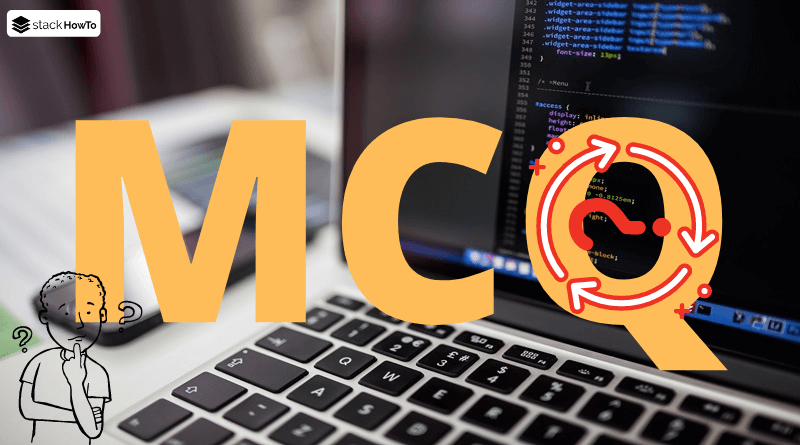MCQ

# Java MCQ – Multiple Choice Questions and Answers – Data Types and Variables – Part 1

This collection of Java Multiple Choice Questions and Answers (MCQs): Quizzes & Practice Tests with Answer focuses on “Java data types and variables”.

###### 1. Java is a _________ language.

A dynamically typed

B weakly typed

C strongly typed

D medium typed

C
Java is a strongly typed programming language since each variable must be declared with a data type. A variable can’t get started without knowing the range of values it can contain, and once it is declared, the data type of the variable can’t change.

###### 2. How many primitive data types are there in Java programming?

A 2

B 3

C 5

D 8

D
Primitive types are the most fundamental data types accessible in Java. There are 8 primitive data types: int, float, boolean, byte, char, short, long and double.

###### 3. Size of int in java is ______

A 16 bit

B 32 bit

C 64 bit

D Relies on the execution environment

B
Size of int in java is 4 bytes = 2^4 = 32 bit.

###### 4. Which is the smallest integer data type?

A int

B byte

C short

D long

B
The smallest integer data type is byte. Example:

```System.out.println("Size of int: " + (Integer.SIZE/8) + " bytes.");
System.out.println("Size of byte: " + (Byte.SIZE/8) + " bytes.");
System.out.println("Size of short: " + (Short.SIZE/8) + " bytes.");
System.out.println("Size of long: " + (Long.SIZE/8) + " bytes.");```

Output:

```Size of int: 4 bytes.
Size of byte: 1 bytes.
Size of short: 2 bytes.
Size of long: 8 bytes.```

###### 5. The smaller integer type is _______ and its size is __ bits.

A byte, 8

B byte, 1

C short, 8

D int, 4

A
The smaller integer type is byte and its size is 8 bits (1 byte = 8 bits).

###### 6. Which is not a primitive data type in Java ?

A float

B enum

C double

D int

B
enum is not a primitive data type in Java

###### 7. Integer Data type doesn’t incorporate following primitive data type ______.

A short

B byte

C long

D double

D
Integers includes following primitive data type: byte, short, int, and long.

###### 8. Which data types go through floating data types ?

A int

B byte

C long

D double

D
Floating-point numbers includes float and double.

###### 9. Character data type can’t store _______ value.

A Special Character

B Digit

C String

D Letter

C
Character data type can’t store String value.

###### 10. What is the size of integer in Java?

A 1 Bytes

B 2 Bytes

C 4 Bytes

D 8 Bytes

C
The size of integer in Java is 4 Bytes.MCQPractice competitive and technical Multiple Choice Questions and Answers (MCQs) with simple and logical explanations to prepare for tests and interviews.Read More